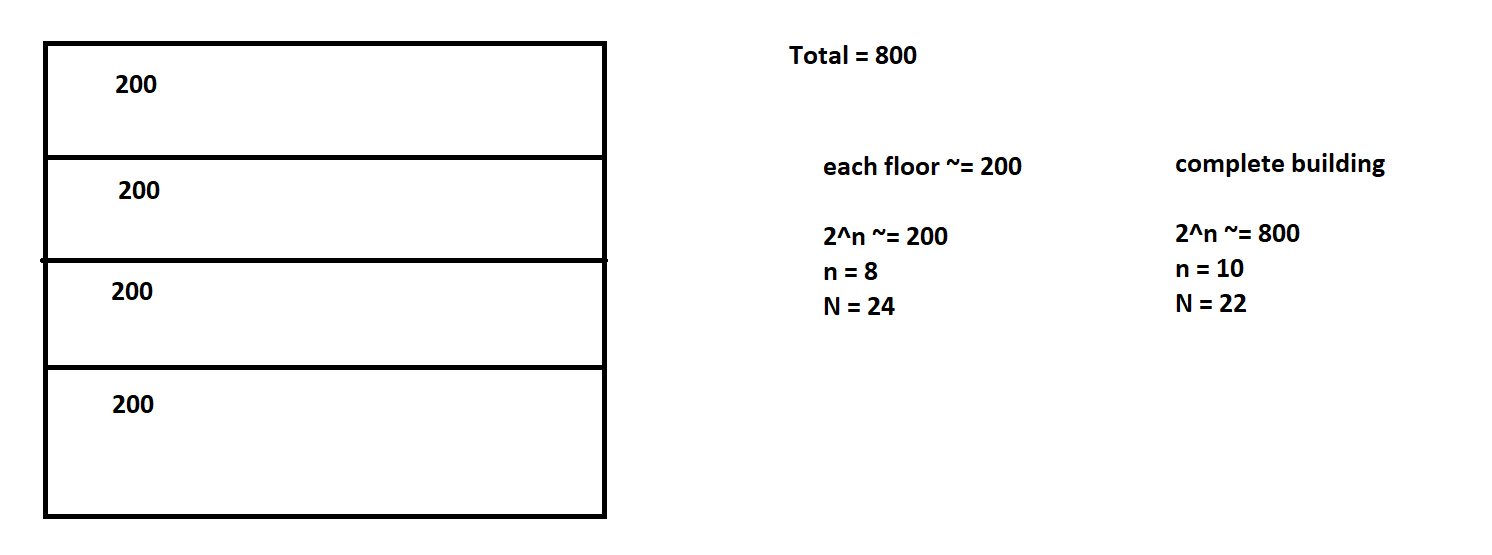## Private Network vs Public Network

• Public Network: A network which can be accessed from internet
• Private Network: A network which cannot be accessed directly from internet.
• For private networks there are some predefined or reserved cidr ranges• CIDR Ranges
• 10.0.0.0/8
• 172.16.0.0/12
• 192.168.0.0/16

## Subnet

• Network can be divided into multiple smaller networks called as subnets

### Consider a building with 4 floors, we need to design a network

• Each floor requires around 200 devices to be connected
• Total building around 800 devices• for building N = 22
``````building network cidr: 192.168.0.0/22

each floor N = 24

building SM: 11111111.11111111.11111100.00000000
floor SM:    11111111.11111111.11111111.00000000
-------------------------------------
xx
00 => 192.168.0+(0).0/24
01 => 192.168.0+(1).0/24
10 => 192.168.0+(2).0/24
11 => 192.168.0+(3).0/24
192.168.0.0/24
192.168.1.0/24
192.168.2.0/24
192.168.3.0/24
``````
• Each floor requires around 20000 devices to be connected
``````each floor ~= 20000
2^n ~= 20000
n = 15
N = 17

building ~= 80000
2^n ~= 80000
n = 17
N = 15

building => x.x.x.x/15 => 172.16.0.0/15

bd SM: 11111111.11111110.00000000.00000000
fl SM: 11111111.11111111.10000000.00000000
---------------------------------------
x.x
0.0  => 172.(16+0).0.0/17 => 172.16.0.0/17
0.1  => 172.(16+0).128.0/17 => 172.16.128.0/17
1.0  => 172.(16+1).0.0/17 => 172.17.0.0/17
1.1  => 172.(16+1).128.0/17 => 172.17.128.0/17
``````
• Consider building has 8 floors, each floor requires 60 devices
``````floor ~= 60
2^n ~= 60
n = 6
N = 26

building = 480
2^n ~= 480
n = 9
N = 23
10.0.0.0/23

bd SM:  11111111.11111111.11111110.00000000
fl SM:  11111111.11111111.11111111.11000000
-------------------
x.xx
0.00  => 10.0.0.0/26
0.01  => 10.0.0.64/26
0.10  => 10.0.0.128/26
0.11  => 10.0.0.192/26
1.00  => 10.0.1.0/26
1.01  => 10.0.1.64/26
1.10  => 10.0.1.128/26
1.11  => 10.0.1.192/26
``````
• Consider a building with 8 floors and each floor requires 25000 devices
``````floor ~= 25000
2^n ~= 25000
n = 15
N = 17

building ~= 200000
2^n ~= 200000
n = 18
N = 14

10.0.0.0/14

bd SM: 11111111.11111100.00000000.00000000
fl SM: 11111111.11111111.10000000.00000000
-------------------------------------
xx.x
00.0   => 10.0.0.0/17
00.1   => 10.0.128.0/17
01.0   => 10.1.0.0/17
01.1   => 10.1.128.0/17
10.0   => 10.2.0.0/17
10.1   => 10.2.128.0/17
11.0   => 10.3.0.0/17
11.1   => 10.3.128.0/17
``````

This site uses Akismet to reduce spam. Learn how your comment data is processed.